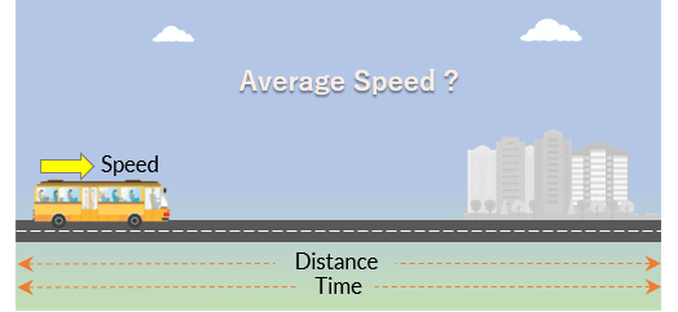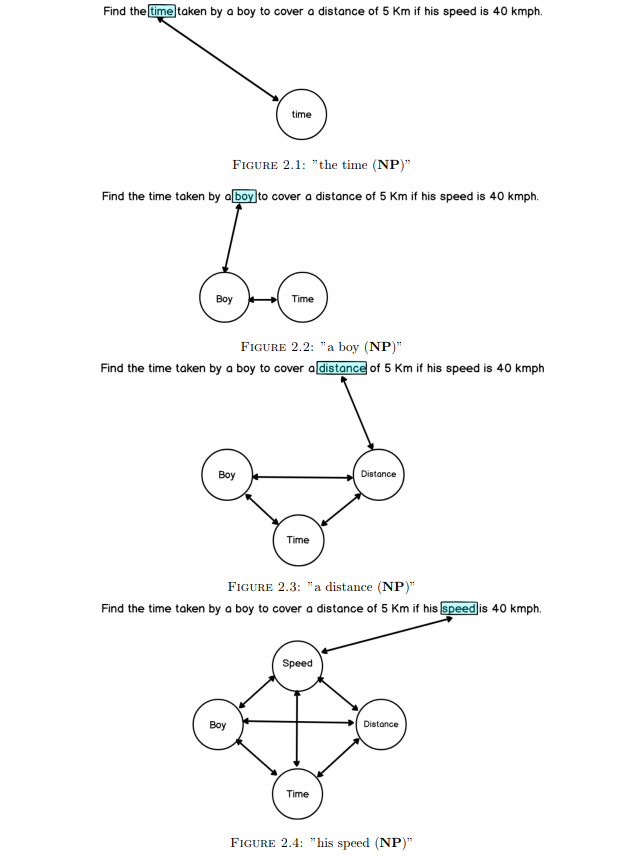# Word Problem Solver### Problem Statement

Given a preliminary word problem based on speed, time and distance relationship in natural language, generate a step-by-step solution for it

### Proposed Solution

Our approach to solve mathematical word problems is based on the ability of represent the problem via relationships between various object described in the text. We start out by creating a graph which represents the hypothetical world described in the problem with a global function space defining the rules/association between different types of objects.

We keep on adding nodes in the graph as when a new object (Noun Phrase) appears in the text and depict the association between two objects by an edge between them. If multiple functions are there multiple types of edges need to be created. If a Noun Phrase is an anaphora, all the objects which would have been linked to this noun phrase would instead be linked with the representative mention and node corresponding to the current Noun Phrase won’t be added to the graph.

A set of nodes are eligible as arguments for a function iff they correspond to the object types used in the functional relationship and are connected by edges corresponding to the function. Each measurable quantity has a value attribute associated to it which stores the value of that quantity. Once the graph is generated, we need to calculate the value of the query node. To do this, we traverse the graph starting DFS at query node (Top-Down approach) and check if we are able to calculate its value along any one of the computation path. We work recursively to evaluate all the nodes of the computation path from leaf and then finally evaluate the value of the root node. The following figures explain the graph construction.The implimentation details are available in project report.

### Testing

The problems that it could understand were restricted and testing needed a controlled environment. The biggest challenge that we faced was handling prior world knowledge in the system. Currently, we only took those problem which didn’t need this world-knowledge for evaluation. This assumption seems a bit heavy so, we came up with an approach to incorporate it and have left it under future work for testing and validating its effectiveness.

We also assumed that the problems didn’t need the knowledge of monotonic nature of the quantities and the association of words to modifications in the values of quantities like Late w.r.t time refers to addition of time to certain time object similarly longer w.r.t distance implies higher magnitude.

Owing to a deterministic algorithm for query solving, if the system was able to understand the language it gives the correct answer. So, we took a set of 11 different type of problems that needed only Distance = Speed*Time as relationship. Out of these we were able to solve 7 problems some of which are mentioned below:

1. How much time Raman will take to cover a distance of 400 km if he runs at a speed of 20 kmph ?
2. The distance between two stations is 240 km. A train takes 4 hours to cover that distance. Calculate the speed of the train.
3. A person runs a distance of 60 km in 5 hours. What is his speed ?
4. Nancy travelled a distance of 455 km by car in 10 hours. Find the speed of the car.
5. Ramesh travels at a speed of 30 kmph for a time of 2 hours to travel a certain distance. How much time will he take to cover that distance if his new speed is 20 kmph?
6. If it takes 3 hours to drive a distance of 192 km on a motorway, what would be your speed?

We propose the following methods to overcome the challenges mentioned earlier and would be following our future work in this direction to improve the system:

1. Automating the graph generation for any type of problem

• Graph Neural Nets are highly effective in handling word to graph manipulations. They should work out better in handling linking between different objects.
• The monotonic and sequential nature of the quantities can be derived using Semantic Role Labeller. This would be helpful in adding word-to-quantity manipulations in general.
2. Synonymous descriptions like associating length to distance, age to time can be worked by training a ML classifier to associate these labels to noun-phrases. Semantic similarity or paraphrase matching systems might work out better in these.

3. There are some relationships which are not given by function space but rather by ownership like a distance travelled by a car is owned by the agent(car). Such relationships can help in handling ambiguity in associating quantities together.